Math Calculators, Lessons and Formulas

It is time to solve your math problem

mathportal.org
• Pre algebra
• Whole numbers
• The Number Line

# The Number Line

ans:
syntax error
C
DEL
ANS
±
(
)
÷
×
7
8
9
4
5
6
+
1
2
3
=
0
.
auto next question
calculator
•  Question 1: 1 pts On the number line, point $B$ has coordinate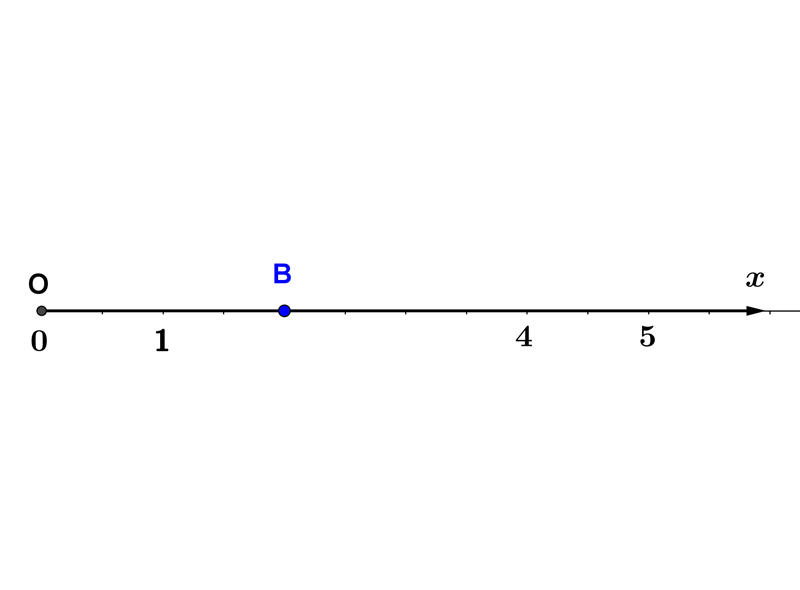$3$ $2$ $1$ $0$
•  Question 2: 1 pts Each whole number corresponds to exactly one point on the number line.
•  Question 3: 1 pts On the number line, the coordinate of point $C$ is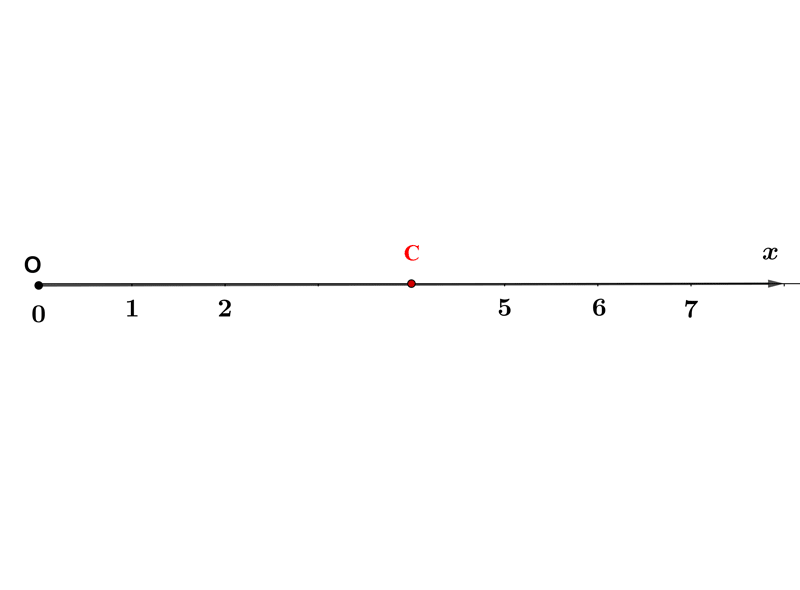3 4 5 6
•  Question 4: 1 pts The origin on the number line has coordinate
 1 2 3 0
•  Question 5: 2 pts The measure of the distance between point $A(2)$ and $B(8)$ is the:
 $6$ $8$ $2$ $5$
•  Question 6: 2 pts Use the number line to find the following measure.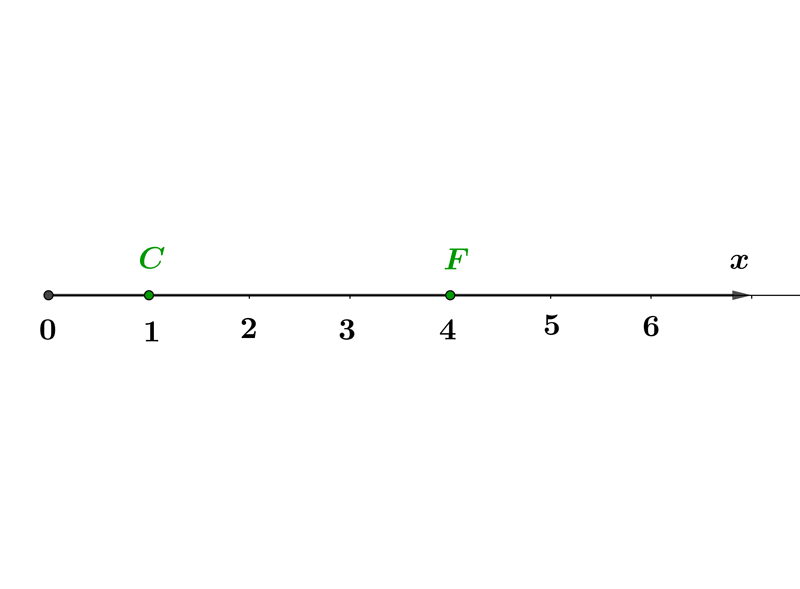$CF=$
•  Question 7: 2 pts Which point corresponds to the greatest whole number?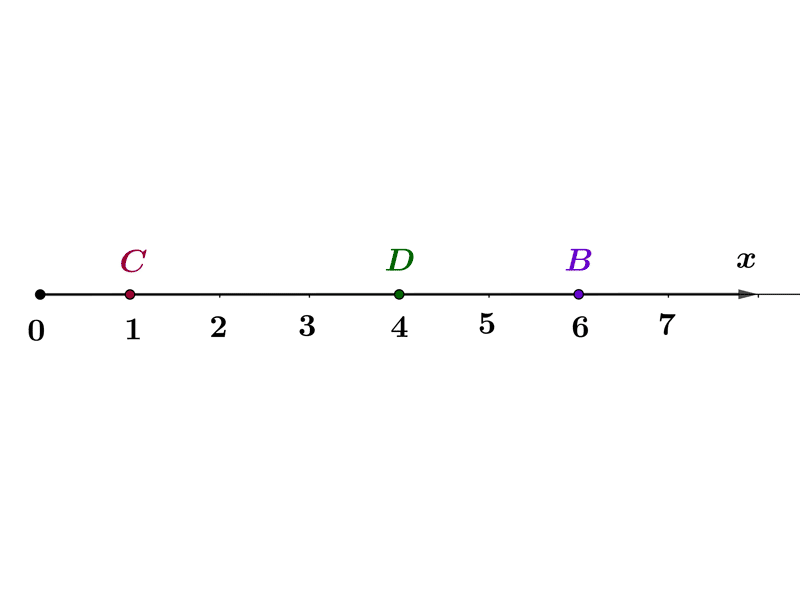The point
•  Question 8: 2 pts Every whole number has a predecessor.
•  Question 9: 3 pts Use the number line and describe the subset of the whole numbers that the inequality represent $-2\leq x<4.$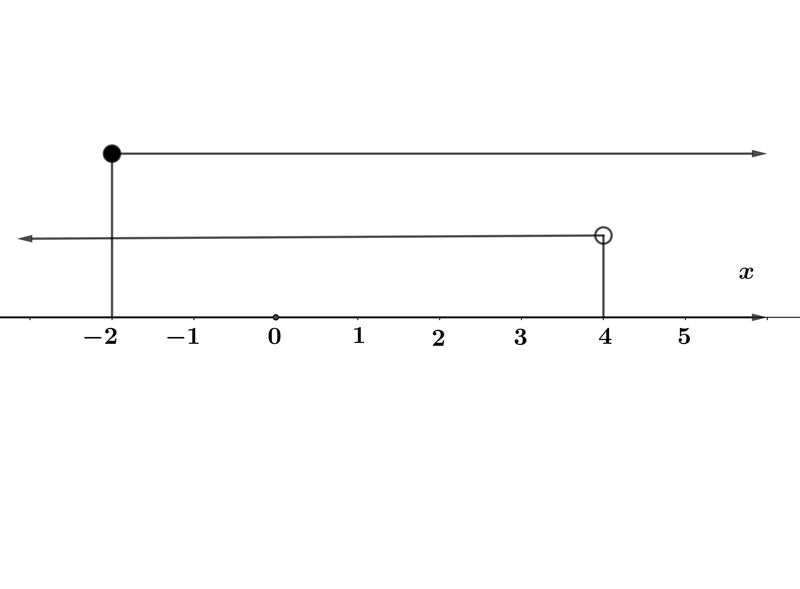$x\in\{-2,-1,0,1,2,3,4\}$ $x\in\{0,1,2,3,4\}$ $x\in\{0,1,2,3\}$ $x\in\{-1,0,1,2,3,4\}$
•  Question 10: 3 pts Use the number line and describe the subset of the whole numbers that the inequality represent $-5 \leq x\leq 5$.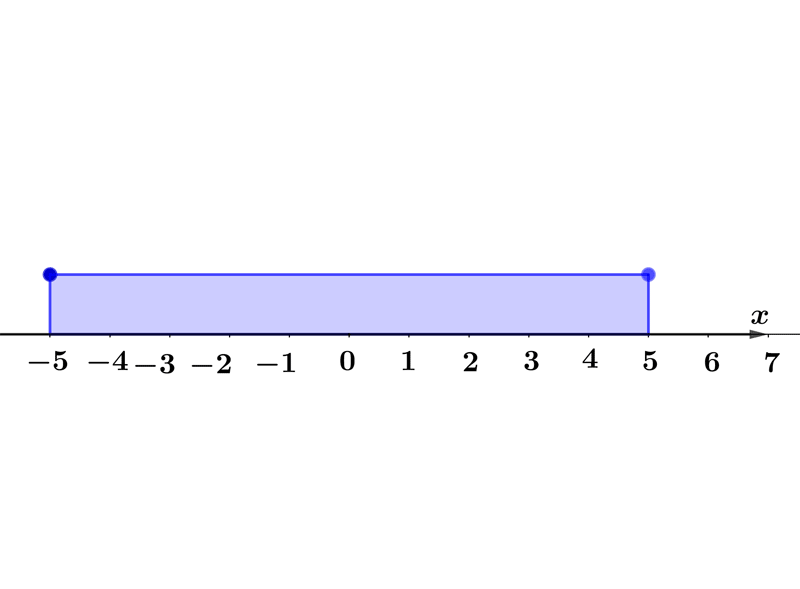$x\in\{-5,0,5\}$ $x\in\{0,1,2,3,4,5\}$ $x\in\{1,2,3,4,5\}$